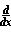Home    |    Teacher    |    Parents    |    Glossary    |    About UsEmail this page to a friendResources· Cool Tools · Formulas & Tables · References · Test Preparation · Study Tips · Wonders of MathSearchProof: Sum Rule (Math | Calculus | Derivatives | Identities | Sum Rule)[f(x) + g(x)] =f(x) +g(x)

Proof of[f(x) + g(x)] =f(x) +g(x) from the definition

We can use the definition of the derivative:f(x) = lim d-->0 f(x+d)-f(x) d
Therefore,[f(x) + g(x)] can be written as such:[f(x) + g(x)] =
 lim d-->0 [f(x+d)+g(x+d)] - [f(x)+g(x)] d
 = lim    d-->0 ( [f(x+d)-f(x)] d + [g(x+d)-g(x)] d )
 = lim    d-->0 f(x+d)-f(x) d
+ lim
d-->0
g(x+d)-g(x)
d